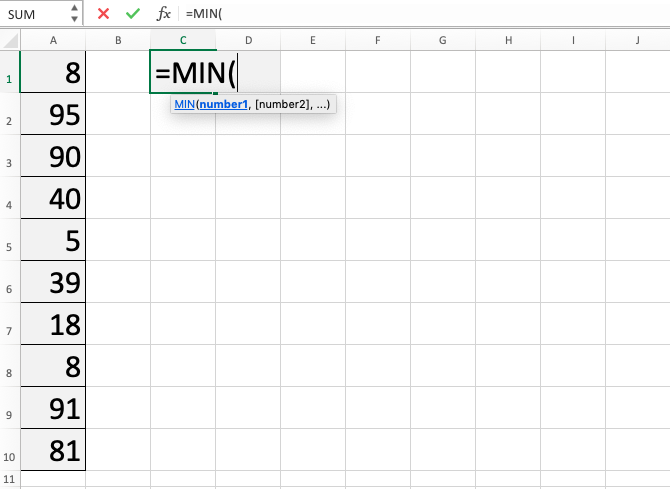MIN Function in Excel - Compute Expert

# MIN Function in Excel

In this tutorial, you will learn about how to use excel MIN function. This formula can be used to get the smallest value in a group of numbers that we have in this spreadsheet tool.

## Why We Need to Learn About Excel MIN Function?

In doing digit data processing on spreadsheet software, often we need to find the smallest value from a group of numbers that we have.

For example, when we want to know the production priority of our company factory based on the smallest demand of a product kind or get the knowledge of a region with the lowest sales performance for a company product. For these two cases, we need a way to find the smallest value from all products’ production demand in the first case and from the sales numbers of all regions where the company sells that product for the second case. Of course, if the data we have is much, then it becomes difficult to do the process manually.

There is MIN function in excel which can be used to help to find the smallest numeric data in a group of numbers fast and accurate. If we often need to look for a digit based on the rank it has on this spreadsheet software, then excel MIN function usage can be an essential thing to be understood.

## What is MIN Function in Excel?

MIN function in excel is a formula to look for the smallest value in a group of numbers that we have. This formula is one of the formula with the result based on ranking on this spreadsheet tool besides MAX and RANK.

Brief explanation about the inputs in this formula is as follows:

=MIN(number1, number2, …)

Note:
number1, number2, … = all the numbers that you want to find the smallest value of

## How to Use Excel MIN Function?

The following will explain in detail how to use MIN function in excel. Excel MIN function just needs the input of all the digits to be looked at and will return their smallest value for us. This MIN function in excel is pretty easy to use and similar on its writing with the MAX formula.

## Using MIN Function in Excel

1. Type equal sign ( = ) in the cell where you want to put the result in2. Type MIN (can be with large and small letters) and open bracket sign after =3. Drag cursor from the most top left to the most bottom right on the cells where you want to find the smallest value from. You can also type comma sign ( , ) and add cell range in another place until all of them are in the formula4. Type close bracket sign after all cells that you want to be processed have already been inputted5. Press Enter
6. The process is done!## Exercise

After learning the usage steps from the tutorial above, you can practice your understanding by doing this exercise!

### Questions

1. What is the first row’s smallest numeral?
2. What is the second row’s smallest numeral?
3. What is the third row’s smallest numeral?

• In using this formula, drag cell cursor and numbers or cell coordinates input with comma sign separation can be done in one writing
• If you want, cell coordinates which are near to each other for excel MIN function input can also be written manually separated for the most top-left cell and most bottom-right cell by two dots sign ( : ) (Writing example: B1:D25)
• There are other formulas to find ranking related data in this spreadsheet software like MAX and RANK. If you want to learn them too, then you can look at the tutorials from other parts of this Compute Expert blog!

Get updated excel info from Compute Expert by registering your email. It's free!